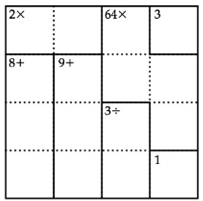Chapter 1.1, Problem 3EEMathematical Excursions (MindTap C...

4th Edition
Richard N. Aufmann + 3 others
ISBN: 9781305965584

Solutions

Chapter
SectionMathematical Excursions (MindTap C...

4th Edition
Richard N. Aufmann + 3 others
ISBN: 9781305965584
Textbook Problem

EXCURSION EXERCISESSolve each of the, following puzzles. Note: The authors of this textbook are not associated with the KenKen brand. Thus the following puzzles are not official KenKen puzzles; however, each puzzle can be solved using the same techniques one would use to solve an Official KenKen puzzle.To determine

The solution of the given puzzle.

Explanation

Given information:

Concept Used:

• As per the kenken rules of puzzles the numbers that can be used for 4×4 puzzles are 1,2,3,and4 . A 6×6 puzzle may use numbers 1 through 6.
• Also each box of the puzzle must contain a required number. If there is more than one box then target is found using the arithmetic operations.

Calculation:

The given puzzle is

Now apply the above mentioned concept.

The above box/cage can be highlighted as below

The target boxes can be labeled as shown below

The target box will be filled as per the rules of kenken puzzle rules as shown below:

Still sussing out bartleby?

Check out a sample textbook solution.

See a sample solution

The Solution to Your Study Problems

Bartleby provides explanations to thousands of textbook problems written by our experts, many with advanced degrees!

Get Started

Given that the point (2,4) lies on the line 2x+ky=8, find k.

Finite Mathematics for the Managerial, Life, and Social Sciences

Simplify: j3

Elementary Technical Mathematics

In Exercises 1-6, simplify the expression. 4. x3+2x23x2x2x+3

Applied Calculus for the Managerial, Life, and Social Sciences: A Brief Approach

In Exercises 516, evaluate the given quantity. log22

Finite Mathematics and Applied Calculus (MindTap Course List)

Simplify each radical expression. 22+112

Trigonometry (MindTap Course List)